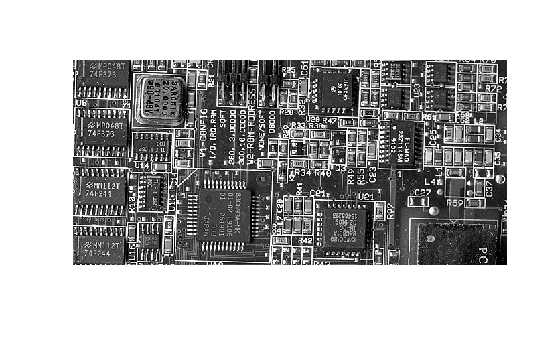Main Content

# Derive Statistics from GLCM and Plot Correlation

This example shows how to create a set of GLCMs and derive statistics from them. The example also illustrates how the statistics returned by graycoprops have a direct relationship to the original input image.

Read an image into the workspace and display it. The example converts the truecolor image to a grayscale image and then, for this example, rotates it 90 degrees.

```circuitBoard = rot90(rgb2gray(imread('board.tif'))); imshow(circuitBoard)```Define offsets of varying direction and distance. Because the image contains objects of a variety of shapes and sizes that are arranged in horizontal and vertical directions, the example specifies a set of horizontal offsets that only vary in distance.

`offsets0 = [zeros(40,1) (1:40)'];`

Create the GLCMs. Call the `graycomatrix` function specifying the offsets.

`glcms = graycomatrix(circuitBoard,'Offset',offsets0);`

Derive statistics from the GLCMs using the `graycoprops` function. The example calculates the contrast and correlation.

`stats = graycoprops(glcms,'Contrast Correlation');`

Plot correlation as a function of offset.

```figure, plot([stats.Correlation]); title('Texture Correlation as a function of offset'); xlabel('Horizontal Offset') ylabel('Correlation')```The plot contains peaks at offsets 7, 15, 23, and 30. If you examine the input image closely, you can see that certain vertical elements in the image have a periodic pattern that repeats every seven pixels.

Download ebook